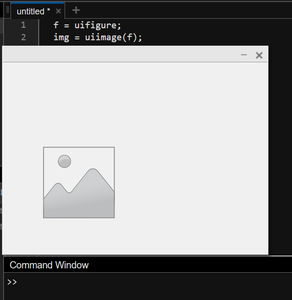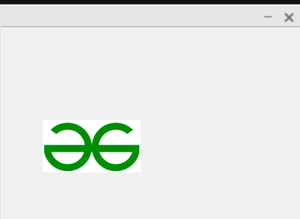GFG App
Open AppBrowser
Continue

# How to Create an Image Component in MATLAB?

MATLAB is an extensive tool that provides various options to its users. Creating image components is one of those tools. MATLAB provides simple functions to create image components. The uiimage function creates a new image component in a new figure by calling on the uifigure function. Usage of uiimage function.

Example 1:

## Matlab

 `% MATLAB Code for image component ` `f = uifigure; ` `img = uiimage(f);`

Output:

This code snippet creates a new figure which can further be used to store images later on. It’ll show an empty figure as follows:Example 2

Now, let us add an image to this figure.

## Matlab

 `% MATLAB Code ` `f = uifigure; ` `img = uiimage(f); ` ` `  `% This takes the image "GFGlogo.jpg" ` `img.ImageSource = ``"GFGlogo.jpg"``;`

Output:

This new image component can be used as an image, logo, etc. in a MATLAB application.A simple usage of these image components is by using to open a link when clicked. See the following snippet for a better understanding.

Example 3:

## Matlab

 `% MATLAB Code for image insertion ` `f = uifigure; ` `img = uiimage(f); ` `img.ImageSource = ``"YVkEGi.jpg"``; ` ` `  `% Adding url to image ` `img.URL = ``"https://www.geeksforgeeks.org/"``; ` ` `  `% Text displayed when hovering over the image ` `img.Tooltip = ``"Go to GeeksForGeeks"``;`

Output:

This will create an image with a hyperlink:### Conclusion:

This article discussed how to create image components in MATLAB and some of its usage.

My Personal Notes arrow_drop_up